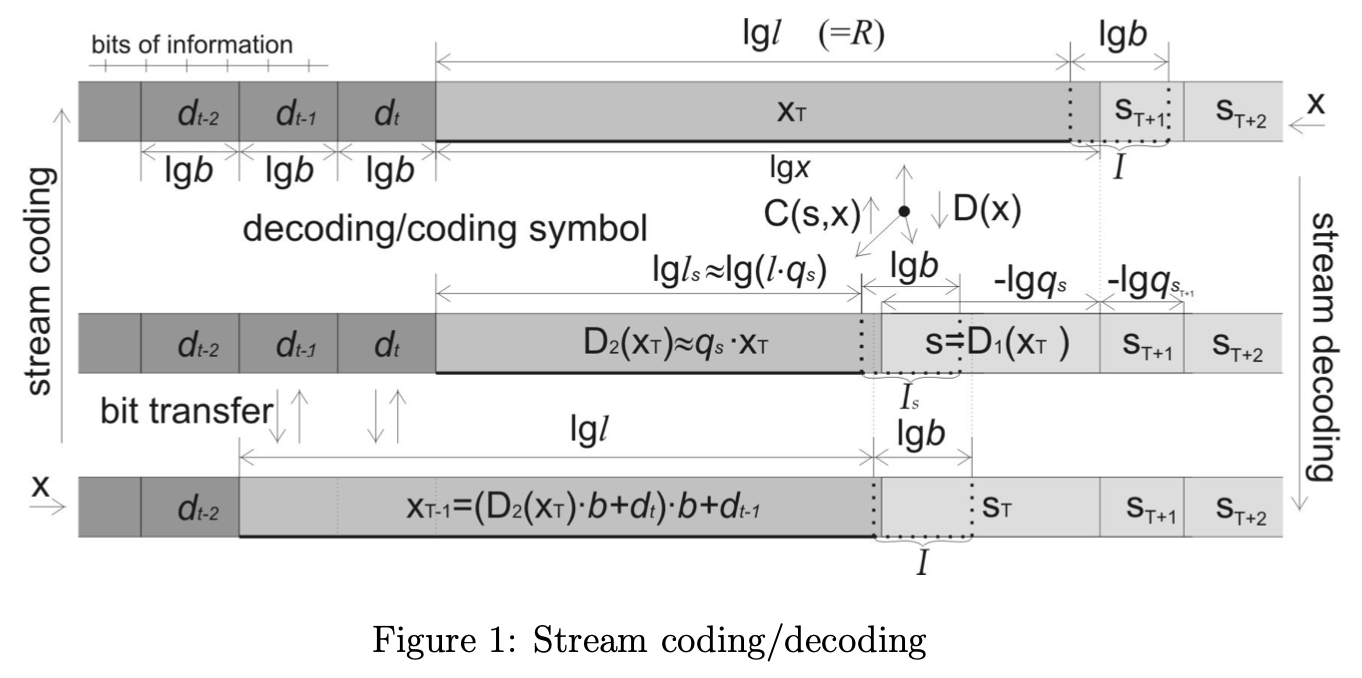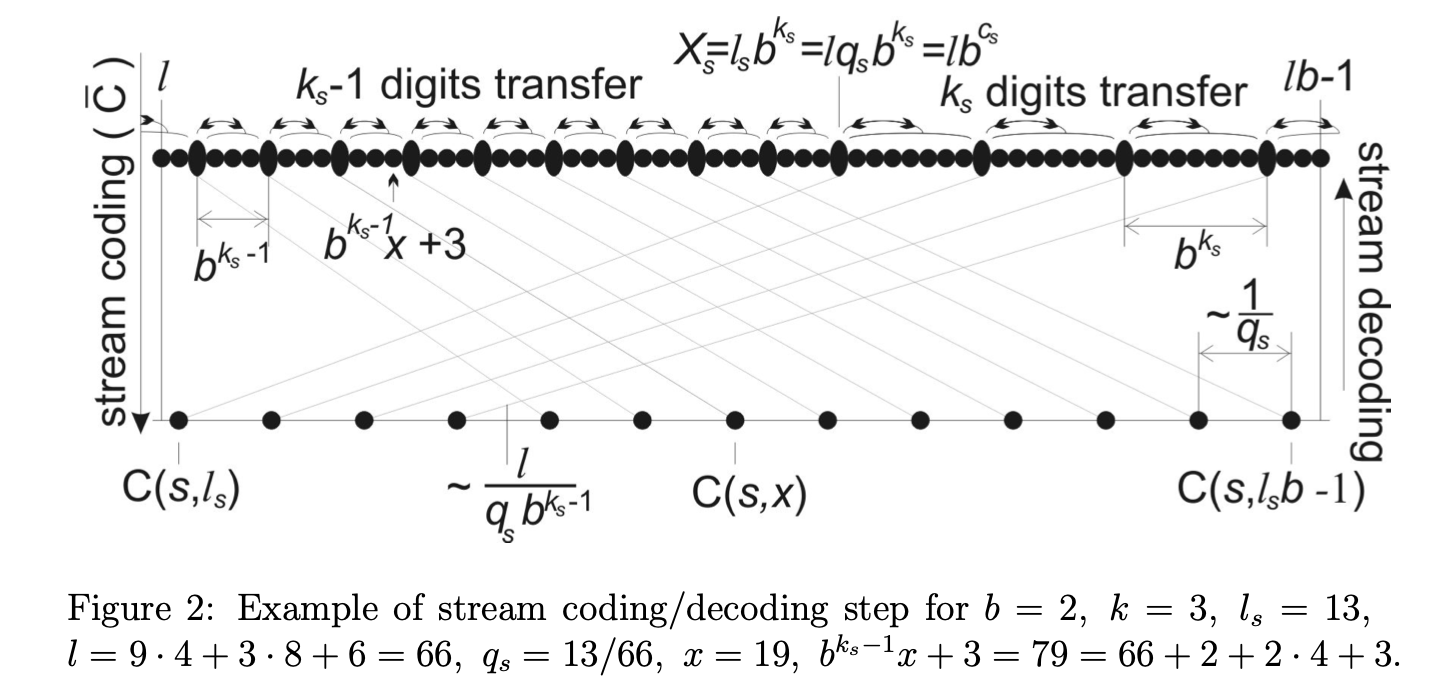Graph all the things

analyzing all the things you forgot to wonder about

# Streaming Asymmetric Numeral System Explained

2023-09-10
interests: compression (explained for beginners)

A few months ago, I spent several hours struggling to understand the inner workings of a lossless compression technique called Tabled Asymmetric Numeral System (tANS). I couldn't find any good* explanations for the leap involved from vanilla ANS to streaming ANS, so I vowed to create one.

### What are we compressing?

Symbols!

As a silly example, say we want to compress the words "freer reef referee referrer" where each letter is a symbol (ignoring spaces). There are 3 distinct symbols - "e", "f", and "r" (an alphabet size of 3), and 24 symbols total.

In practice, symbols have a slightly higher-level meaning. The classic DEFLATE format (e.g. gzip) uses 288 distinct symbols: 256 for all literal bytes, 29 meaning "look back at earlier symbols and copy some", 1 for the end of the data block, and 2 for unimplemented purposes. Pco compresses numbers by binning them, and it represents each bin as a symbol.

### How are we compressing the symbols?

Turning them into a really big number!

Well, technically two numbers: one regular-size number, say up to, encoding the total number of symbols, followed by the really big number. In this way we can spendbits to compress the data. If the compressed data ends up being a gigabit,will end up being about.

### Vanilla ANS

If a symbol has frequencyin the data, we hope to spend aboutbits to encode the character. For example, if we have 2 equally frequent symbols, we expect to spend about 1 bit per symbol. If we have 8 equally frequent symbols, we expect to spend about 3 bits per symbol. In practice, each symbol will have its own arbitrary frequency, so we'd like to spend more bits per uncommon symbol and fewer bits per common one.

We start by making a small index of symbols approximately mimicking the frequency of each symbol. In our silly example above, we might choose an index of "eefr" since "e" is a bit more common than the other symbols. Then we tile it infinitely along the number line:Vanilla ANS's compression algorithm is dead simple: start with, and when we encode theth symbol, letbe the index at which thest instance of that symbol occurs. We use 0-based indexing so that the 0th instance really means the first. The finalis our really big number.

Let's do an example, compressing the first 2 symbols "f" and "r". Sinceand the first symbol is "f", we set, where the 0th (first) "f" appears in the index:Then, sinceand the second symbol is "r", we setwhere the 2nd (third) "r" appears in the index:It's also simple to decode: starting from, iteratively decode the symbol atand letbe the number of times that symbol appeared in the table up to (but not including). In the example above, we can start from, see that 11 corresponds to an "r", and infersince 11 is the third instance of an "r". Then we can useto infer the previous symbol was "f" and so forth.

Since half the symbols in our index are "e", each time we encode an "e" we roughly double, adding one bit. And each time we encode an "f" or "r" we roughly quadruple, adding two bits. With a larger index, we could get closer to the ideal number of bits (per "e", 2.58 per "f", and 1.43 per "r").

To summarize vanilla ANS in a single diagram:### Streaming and normalization

Vanilla ANS is great and easy to understand, but it's impractical because it involves arithmetic on an extremely large integer. To make it faster and more memory-efficient, we need a way to keepsmall. We do this by adding a step to encoding called normalization, and a corresponding step to decoding called denormalization (sometimes these are both called "renormalization", but that seems redundant and ambiguous to me):Normalization and denormalization keepin a manageable range by writing bits out early instead of lettinggrow arbitrarily large. In particular, we require the index sizeto be a power of 2, and we keepin the interval. Emitting bits early like this sacrifices a small amount information during normalization, hurting compression ratio a smidgen, but spares us from doing impractical arithmetic.

Here's the main thing that tripped me up learning streaming ANS: I thought it would be equivalent to a special case of vanilla ANS with a-sized index. This is not the case. Normalization has no vanilla ANS analog, and it reduces the precision of, regardless of index size.

To normalizewhen compressing a symbol, we count, the number of times the symbol appears in the index, then find the exponentsuch that. We write out the least significantbits ofto the bitstream, then returnas the value ofnormalized to the symbol. Normalization is complete, and we then apply the vanilla ANS step to, giving.

If you think about it,must be in the interval, since it's the index of theth occurrence of the symbol in the index andis between 1- and 2-times the number of occurences of the symbol in the index.

Denormalization is just the opposite. After undoing vanilla ANS, we get bothand the symbol, and then we can find the exponentsuch that. We read thoseleast significant bits back from the bitstream and get.

Normalization isn't magic, but it satisfies 3 important properties:

• It's invertible. (necessary to get the symbols back)
• It returns ansuch that vanilla ANS applied tois in a fixed range. (improves compression/decompression speed and memory usage)
• It carries the most significant information frominto. (doesn't hurt compression ratio too much)

### Range Asymmetric Numeral System (rANS)

This is just a name for the streaming algorithm described in the previous section. Done!

### Tabled Asymmetric Numeral System (tANS)

Finding the value ofused during normalization and denormalization on the fly can be expensive. It also affects the number of bits you need to write/read, so CPUs need to block on evaluatingbefore evaluating the next steps. It can be faster to precompute the information needed for allstates of the index.

For instance, in the decoder, we can make a table from everyin the normalized rangeto

• its symbol,
• how many more bits to read for denormalization,
• and the next value ofto add the least significant bits to.

This is equivalent to rANS, but is usually faster when the size of the data is much larger than the index.

### Confusing thing explained: decoding doesn't have enough options?

In our "eefr" example with streaming, "e" has 2 occurrences in index of size 4, so every time we denormalize after an "e" during decoding we read exactly 1 bit. So, based on the bitstream, the decoder is choosing between at most 2 symbols to decode next! What if the next symbol is supposed to be the 3rd one though? If we had swapped that next symbol with the 3rd one during compression, how could the decoder possibly decode it?

The trick is that compression was done in the opposite order. It's exactly like the movie Tenet (wait a sec, that won't help anyone understand...). To the decoder, we're talking about the next symbol, but to the encoder, it's the previous symbol. If we swap theth symbol out forduring compression, we'd end up in a different statethat does have the option to decode.

One way of thinking about it: the encoder and decoder each encounter a choice at every symbol. The compressor always has exactly one option per symbol in the alphabet, but the decoder may have more or fewer. Notice that every statehas an out-edge (encoding option) of each symbol, but doesn't necessarily have in in-edge (decoding option) for each symbol: